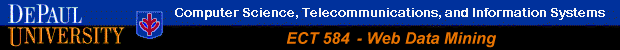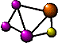Association Rule Mining with WEKA

 The following guide is based WEKA version 3.4.1. Newer versions of WEKA have some differences in interface, module structure, and additional implemented techniques. In this example we focus on the Apriori algorithm for association rule discovery which is essentially unchanged in newer versions of WEKA. Additional resources on WEKA, including sample data sets can be found from the official WEKA Web site.

This example illustrates some of the basic elements of associate rule mining using WEKA. The sample data set used for this example, unless otherwise indicated, is the "bank data" described in (Data Preprocessing in WEKA). In this case, our starting point is the discretized data obtained after performing the preprocessing tasks. Figure a1 shows the WEKA explorer interface after opening this data file ("bank-data-final.arff").

Figure a1

Clicking on the "Associate" tab will bring up the interface for the association rule algorithms. The Apriori algorithm which we will use is the default algorithm selected. However, in order to change the parameters for this run (e.g., support, confidence, etc.) we click on the text box immediately to the right of the "Choose" button. Note that this box, at any given time, shows the specific commandline arguments that are to be used for the algorithm. The dialog box for changing the parameters is depicted in Figure a2. Here, you can specify various parameters associated with Apriori. Click on the "More" button to see the synopsis for the different parameters.

Figure a2

WEKA allows the resulting rules to be sorted according to different metrics such as confidence, leverage, and lift. In this example, we have selected lift as the criteria. Furthermore, we have entered 1.5 as the minimum value for lift (or improvement) is computed as the confidence of the rule divided by the support of the right-hand-side (RHS). In a simplified form, given a rule L => R, lift is the ratio of the probability that L and R occur together to the multiple of the two individual probabilities for L and R, i.e.,

lift = Pr(L,R) / Pr(L).Pr(R).

If this value is 1, then L and R are independent. The higher this value, the more likely that the existence of L and R together in a transaction is not just a random occurrence, but because of some relationship between them.

Here we also change the default value of rules (10) to be 100; this indicates that the program will report no more than the top 100 rules (in this case sorted according to their lift values). The upper bound for minimum support is set to 1.0 (100%) and the lower bound to 0.1 (10%). Apriori in WEKA starts with the upper bound support and incrementally decreases support (by delta increments which by default is set to 0.05 or 5%). The algorithm halts when either the specified number of rules are generated, or the lower bound for min. support is reached. The significance testing option is only applicable in the case of confidence and is by default not used (-1.0).

The final selection of parameters for our current run is depicted in Figure a3:

Figure a3

Once the parameters have been set, the commandline text box will show the new command line. We now click on start to run the program. This results in a set of rules as depicted in Figure a4.

Figure a4

The panel on the left ("Result list") now shows an item indicating the algorithm that was run and the time of the run. You can perform multiple runs in the same session each time with different paprmeters. Each run will appear as an item in the Result list panel. Clicking on one of the results in this list will bring up the details of the run, including the discovered rules in the right panel. In addition, right-clicking on the result set allows us to save the result buffer into a separate file. In this case, we save the output in the file bank-data-ar1.txt. A portion of this file is depicted in Figure a5:

Figure a5

Note that the rules were discovered based on the specified threshold values for support and lift. For each rule, the frequency counts for the LHS and RHS of each rule is given, as well as the values for confidence, lift, leverage, and conviction. Note that leverage and lift measure similar things, except that leverage measures the difference between the probability of co-occurrence of L and R (see above example) as the independent probabilities of each of L and R, i.e.,

leverage = Pr(L,R) - Pr(L).Pr(R).

In other words, leverage measures the proportion of additional cases covered by both L and R above those expected if L and R were independent of each other. Thus, for leverage, values above 0 are desirable, whereas for lift, we want to see values greater than 1. Finally, conviction is similar to lift, but it measures the effect of the right-hand-side not being true. It also inverts the ratio. So, convictions is measured as:

conviction = Pr(L).Pr(not R) / Pr(L,R).

Thus, conviction, in contrast to lift is not symmetric (and also has no upper bound).

In most cases, it is sufficient to focus on a combination of support, confidence, and either lift or leverage to quantitatively measure the "quality" of the rule. However, the real value of a rule, in terms of usefulness and actionability is subjective and depends heavily of the particular domain and business objectives.

#### Using the Command Line

In general, using WEKA from the command line provides more flexibility that using the GUI version (we will discuss this more in the context of classification). In the case of association rules, the GUI version does not provide the ability to save the frequent itemsets (independently of the generated rules). We can do this using the command line. If we look at the output of the association rule mining from the above example (the file bank-data-ar1.txt), the actual command line options are given under the "Run information" at the top. In the example, this command line is:

``` weka.associations.Apriori -N 100 -T 1 -C 1.5 -D 0.05 -U 1.0 -M 0.1 -S -1.0 ```

We can use this directly using the "Simple CLI" interface.

In the main WEKA interface, click "Simple CLI" button to start the command line interface. The main command for generating the rules as we did above is:

``` java weka.associations.Apriori options -t directory-path\bank-data-final.arff ```

where the word options is replaced with the command line options, which for the above example are:

``` -N 100 -T 1 -C 1.5 -D 0.05 -U 1.0 -M 0.1 -S -1.0 ```

The additional "-t directory-path\bank-data-final.arff" option tells WEKA to use the file "bank-data-final.arff" as the input file (located in the specified directory). This command will produce exactly the same output as the previous GUI example. However, we can add an additional option ("-I") which results in the generation of all frequent itemsets:

``` java weka.associations.Apriori options -I -t directory-path\bank-data-final.arff ```

This command as it is used in the SimpleCLI interface is depicted in Figure a6:

Figure a6

When ready, press enter to run the program with the indicated options. The result of this command will be displayed in the top panel of the Simple CLI interface. Here, the results have been saved into a file bank-data- ar2.txt. You will notice that before the rules, the output includes itemset of various sizes generated at different iterations of Apriori algorithm (in this case, L1 through L5) along with the support count for each itemset. In the case of L1, these are simply the individual items (attributes) that meet the minimum support threshold.Return to Main Page